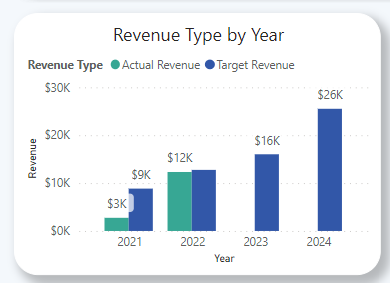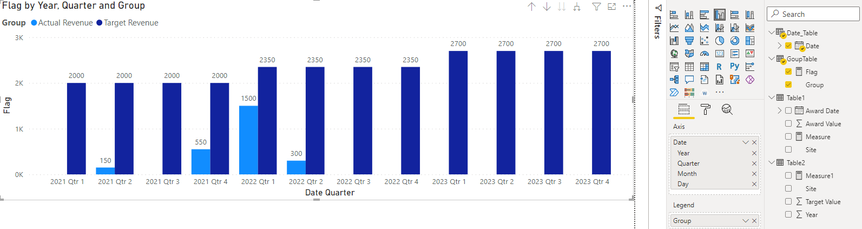cancel
Showing results for
Did you mean:

Fabric is Generally Available. Browse Fabric Presentations. Work towards your Fabric certification with the Cloud Skills Challenge.Helper III

## Calculating Revenue and Targets in one Table

Hello!

I am looking to create a bar chart with a date across the x-axis and then awarded/target values as the two bars (per year).  I need to be able to drill down into each year at least to the Quarter.

Table 1 contains site, award date, and award value while table 2 contains site, target year, and target value. Summarizing the awarded value in table 1 by year is easy enough in a measure but I'm having trouble incorporating the table 2 data.  For the target values, it would be by year, so there needs to be a sum of the target value of all sites by year (in other words, the target value for 2021 is site A + site B + site C + site D, or 2000 and the same thing for 2022, and 2023).

The result would look something like this:Here's some sample data:Thank you!

1 ACCEPTED SOLUTIONCommunity Support

Hi  @JLambs20 ,

Here are the steps you can follow：

1. Create a GroupTable with Enter data.2. Create calculated Date_Table.

``````Date_Table =
CALENDAR(DATE(2021,1,1),DATE(2024,12,31))``````3. Create measure.

``````Measure =
CALCULATE( SUM('Table1'[Award Value]),FILTER(ALL('Table1'),'Table1'[Award Date]>=MIN('Date_Table'[Date])&&'Table1'[Award Date]<=MAX('Date_Table'[Date])))``````
``````Measure1 =
CALCULATE( SUM('Table2'[Target Value]),FILTER(ALL(Table2),'Table2'[Year]>=YEAR(MIN('Date_Table'[Date]))&&'Table2'[Year]<=YEAR(MAX('Date_Table'[Date]))))``````
``````Flag=
SWITCH(
TRUE(),
MAX('GoupTable'[Group])="Actual Revenue",[Measure],
MAX('GoupTable'[Group])="Target Revenue",[Measure1])``````

4. Result:

When the X axis is Year:When Dill down:Best Regards,

Liu Yang

If this post helps, then please consider Accept it as the solution to help the other members find it more quickly

4 REPLIES 4Community Support

Hi  @JLambs20 ,

Here are the steps you can follow：

1. Create a GroupTable with Enter data.2. Create calculated Date_Table.

``````Date_Table =
CALENDAR(DATE(2021,1,1),DATE(2024,12,31))``````3. Create measure.

``````Measure =
CALCULATE( SUM('Table1'[Award Value]),FILTER(ALL('Table1'),'Table1'[Award Date]>=MIN('Date_Table'[Date])&&'Table1'[Award Date]<=MAX('Date_Table'[Date])))``````
``````Measure1 =
CALCULATE( SUM('Table2'[Target Value]),FILTER(ALL(Table2),'Table2'[Year]>=YEAR(MIN('Date_Table'[Date]))&&'Table2'[Year]<=YEAR(MAX('Date_Table'[Date]))))``````
``````Flag=
SWITCH(
TRUE(),
MAX('GoupTable'[Group])="Actual Revenue",[Measure],
MAX('GoupTable'[Group])="Target Revenue",[Measure1])``````

4. Result:

When the X axis is Year:When Dill down:Best Regards,

Liu Yang

If this post helps, then please consider Accept it as the solution to help the other members find it more quicklySuper User

Hi,

Regards,
Ashish Mathur
http://www.ashishmathur.comHelper III

This works when set up the way you mentioned, however, it doesn't allow me to drill down on the award date since we're using the distinct year as the x-axis. Once we've figured that out, I'll be good to go!Frequent Visitor

Hello, @JLambs20 !

I did it this way:

1. Create table with distinct years (DimYear)

2 Create table YearlyAwarded

YearlyAwarded = SUMMARIZE('Table1',[YEAR],"YearlyAward",SUM('Table1'[Award Value]))
3 Create table YearlyTarget:
YearlyTarget = SUMMARIZE('Table2',[Year],"YearlyTarget",SUM('Table2'[Target Value]))
4 Connected all tablesAnnouncements#### Power BI Monthly Update - November 2023

Check out the November 2023 Power BI update to learn about new features.#### Fabric Community News unified experience

Read the latest Fabric Community announcements, including updates on Power BI, Synapse, Data Factory and Data Activator.#### The largest Power BI and Fabric virtual conference

130+ sessions, 130+ speakers, Product managers, MVPs, and experts. All about Power BI and Fabric. Attend online or watch the recordings.Top Solution Authors
Top Kudoed Authors
Users online (2,164)